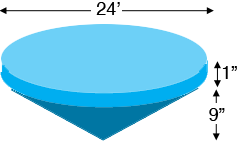SEARCH HOMEMath Central Quandaries & QueriesQuestion from Mike: I have a hole which a 24 ft pool in it is 10" deep in the the centre and goes to 1" inch at the edge want to fill it in with dirt how many yards of dirt would I need to fill it inHi Mike,

You didn't specify the shape of the pool but I assume the surface is a circle of radius 24 feet.I see the hole as a cylinder with base a circle of diameter 24 feet and height 1 inch, and a cone with a base a circle of diameter 24 feet and height 9 inches.

The two important facts here are that the volume of a cylinder is the area of the base tomes the height and the volume of a cone is one third the volume of the cylinder with the same base and height. Thus the volume of the cylindrical top layer is $\pi \; r^2 h$ where $r$ is the radius, 12 feet, and $h$ is the height, 1 inch. The volume of the cone is $\large \frac13 \normalsize \pi \; r^2 h$ where $r$ is again 12 feet but $h$ is 9 inches.

Now you can use our volume calculator. First use it to find the volume of the top layer. Next use it to find the volume of a cylinder with radius 12 feet and height 9 inches and take a third of the result to find the volume of the cone.

I hope this helps,
PennyMath Central is supported by the University of Regina and the Imperial Oil Foundation.